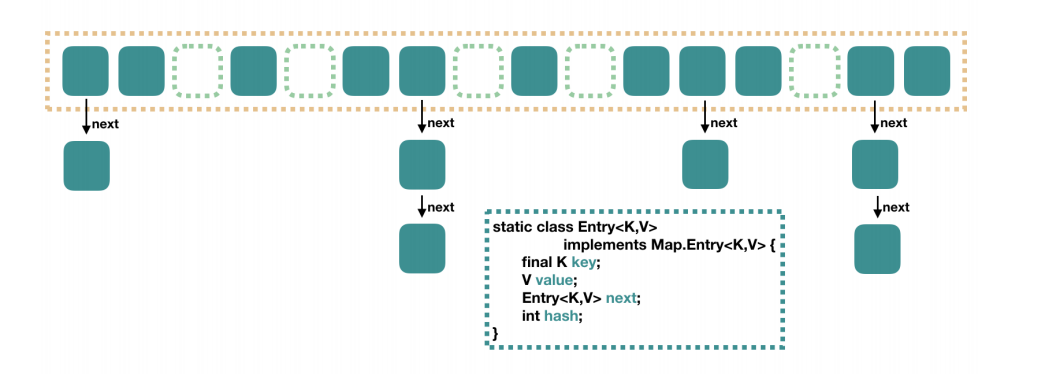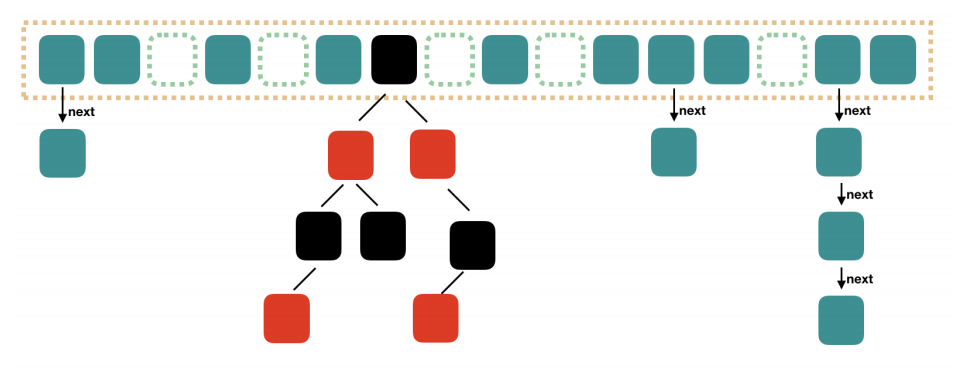# 一文解读所有HashMap的面试题

maxdl

0收藏

#### HashMap

• HashMap 的数据结构
• JDK7 和 JDK8 HashMap哪里不一样
• HashMap是否安全
• HashMap 的扩容机制

#### HashMap 的数据结构

HashMap 里面有几个比较重要的参数：

``````//默认初始容量——必须是2的幂
static final int DEFAULT_INITIAL_CAPACITY = 1 << 4;``````

``````//当没有构造函数中指定使用的负载系数
static final float DEFAULT_LOAD_FACTOR = 0.75f;``````

``````//扩容的阈值，等于 CAPACITY * LOAD_FACTOR
static final int TREEIFY_THRESHOLD = 8;``````

``````//降容的阈值
static final int UNTREEIFY_THRESHOLD = 6;``````

``````//扩容的另外一个参数
static final int MIN_TREEIFY_CAPACITY = 64;``````

`​CAPACITY​`​ 就相当于是 HashMap 中的默认初始容量。

`​LOAD_FACTOR​`​ 负载因子

`​TREEIFY_THRESHOLD​`​ 树化的阈值，也就是说table的node中的链表长度超过这个阈值的时候，该链表会变成树

`​UNTREEIFY_THRESHOLD​`​ 树降级成为链表的阈值（也就是说table的node中的树长度低于这个阈值的时候，树会变成链表）

`​MIN_TREEIFY_CAPACITY​`​ 树化的另一个参数，就是当hashmap中的node的个数大于这个值的时候，hashmap中的有些链表才会变成树。

`​transient Node<K,V>[] table​`​ Hash 表

#### JDK7 和 JDK8 HashMap哪里不一样

JDK7我们大家也都知道，如果按照横向是数组，那么他的纵向每个元素上面，都是一个单向的链表，而横向上，每一个实体，就相当于是一个 Entry 的实例。

• key
• value
• hash值
• 用于单项列表的nextJDK7JDK8

#### HashMap是否安全

``````public V put(K key, V value) {
return putVal(hash(key), key, value, false, true);
}

final V putVal(int hash, K key, V value, boolean onlyIfAbsent,
boolean evict) {
Node<K,V>[] tab; Node<K,V> p; int n, i;
//在这里先进行 Hash表的初始化
if ((tab = table) == null || (n = tab.length) == 0)
n = (tab = resize()).length;
//通过 Hash 值计算在 Hash 表中的位置，并将这个位置的元素赋值给P 如果等于空的话创建一个新的 node
if ((p = tab[i = (n - 1) & hash]) == null)
tab[i] = newNode(hash, key, value, null);
else {
Node<K,V> e; K k;
//Hash表的当前的 index 已经存在了元素，向这个元素后追加链表
if (p.hash == hash &&
((k = p.key) == key || (key != null && key.equals(k))))
e = p;
else if (p instanceof TreeNode)
e = ((TreeNode<K,V>)p).putTreeVal(this, tab, hash, key, value);
else {
for (int binCount = 0; ; ++binCount) {
//新建接点，并且追加到列表
if ((e = p.next) == null) {
p.next = newNode(hash, key, value, null);
if (binCount >= TREEIFY_THRESHOLD - 1) // -1 for 1st
treeifyBin(tab, hash);
break;
}
if (e.hash == hash &&
((k = e.key) == key || (key != null && key.equals(k))))
break;
p = e;
}
}
if (e != null) { // existing mapping for key
V oldValue = e.value;
if (!onlyIfAbsent || oldValue == null)
e.value = value;
afterNodeAccess(e);
return oldValue;
}
}
++modCount;
if (++size > threshold)
resize();
afterNodeInsertion(evict);
return null;
}``````

#### HashMap 的扩容机制

``````    public HashMap(int initialCapacity, float loadFactor) {
if (initialCapacity < 0)
throw new IllegalArgumentException("Illegal initial capacity: " +
initialCapacity);
if (initialCapacity > MAXIMUM_CAPACITY)
initialCapacity = MAXIMUM_CAPACITY;
throw new IllegalArgumentException("Illegal load factor: " +
this.threshold = tableSizeFor(initialCapacity);
}``````

``````static final int tableSizeFor(int cap) {
int n = cap - 1;
//  >>>：无符号右移。无论是正数还是负数，高位通通补0。
n |= n >>> 1;
n |= n >>> 2;
n |= n >>> 4;
n |= n >>> 8;
n |= n >>> 16;
return (n < 0) ? 1 : (n >= MAXIMUM_CAPACITY) ? MAXIMUM_CAPACITY : n + 1;
}``````

``````    final Node<K,V>[] resize() {
Node<K,V>[] oldTab = table;
int oldCap = (oldTab == null) ? 0 : oldTab.length; //旧容量
int oldThr = threshold;// 旧的需要扩容的阈值
int newCap, newThr = 0;
if (oldCap > 0) {//如果不是第一次扩容
if (oldCap >= MAXIMUM_CAPACITY) {
threshold = Integer.MAX_VALUE;// 如果容量大于最大值，将阈值设为最大值，这样不会发生下次扩容
return oldTab;
}
// 扩容容量为上一次容量的两倍
else if ((newCap = oldCap << 1) < MAXIMUM_CAPACITY &&
oldCap >= DEFAULT_INITIAL_CAPACITY)
newThr = oldThr << 1; // 下次扩容阈值等于本次扩容阈值*2，因为扩容会扩为原来容量的两倍，所以依然满足 newThr = newCap * loadFactor
}
else if (oldThr > 0) // 第一次扩容，并且用户指定了初始容量
newCap = oldThr; // 扩展的容量为阈值
else {               // 第一次扩容，并且初始容量和加载因子使用的默认值
newCap = DEFAULT_INITIAL_CAPACITY;
}
if (newThr == 0) {  // 如果用户指定了初始容量时，并且是第一次扩容
float ft = (float)newCap * loadFactor;
newThr = (newCap < MAXIMUM_CAPACITY && ft < (float)MAXIMUM_CAPACITY ?
(int)ft : Integer.MAX_VALUE);
}
threshold = newThr;
@SuppressWarnings({"rawtypes","unchecked"})
Node<K,V>[] newTab = (Node<K,V>[])new Node[newCap];// 新的数组
table = newTab;
if (oldTab != null) {
for (int j = 0; j < oldCap; ++j) { //将旧数组数据移动到新数组
Node<K,V> e;
if ((e = oldTab[j]) != null) {
oldTab[j] = null;
if (e.next == null) // 如果还不是链表或红黑树，把数据直接移动到新数组中对应位置
newTab[e.hash & (newCap - 1)] = e;
else if (e instanceof TreeNode) //红黑树时的移动数据
((TreeNode<K,V>)e).split(this, newTab, j, oldCap);
else { // 链表时移动数据
// 原来的key的hash值对应的数组位置可能会发生变化
// 因为在做与操作时，现有的数组长度多了两倍，也就是多了一位的与计算
// 所以，链表或红黑树中的元素可能在原来位置，或者在原来位置 + 原来数组长度 的位置
Node<K,V> loHead = null, loTail = null;
Node<K,V> hiHead = null, hiTail = null;
Node<K,V> next;
do {
....省略不分
}
}
}
}
return newTab;
}``````帖子
视频
声望
粉丝
相关问题
社区精华内容